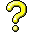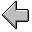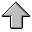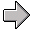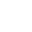Functional Programming6. Streams

## 6.1 Streams - How To Handle Infinite Objects

A stream is a (potentially) countably infinite sequence. The beauty of streams is that they can be processed as lists, but terms of the sequence are only computed when necessary. Streams are sometimes called lazy lists.

In the first implementation, the streams look like lists (thus the `s-first`, `s-rest` operations), but are implemented as dotted pairs, where the first element is the value at the head of the list, and the second is a promise to generate the rest of the list.

code/Streams/stream-primatives.rkt

 `    ``#lang racket` `    `` ` `    ``; based on SICP section 3.5, but shorter and more consistent names` `    `` ` `    ``; implementation of stream primative operations used to implement all additional` `    ``; stream behaviour as defined in the sbase.rkt .  ` `    ``; By redefining these you can have different time/space complexity implementation of streams.` `    `` ` `    ``(provide s-cons)` `    ``(provide s-first)` `    ``(provide s-rest)` `    ``(provide s-empty)` `    ``(provide s-null?)` `    `` ` `    ``; Implementation 1: ` `    ``;   immediate s-first, delayed s-rest, using delay/force` `    `` ` `    ``; streams look like lists (thus the s-first, s-rest operations),` `    ``; but are implemented as dotted pairs, where the first element` `    ``; is the value at the head of the list, and the second is a promise` `    ``; to generate the rest of the list.` `    `` ` `    ``(define-syntax-rule (s-cons a b) (cons a (delay b)))` `    ``(define (s-first s) (car s))` `    ``; force an evaluate of the next term if you want the rest of the stream.` `    ``(define (s-rest s) (force (cdr s)))` `    ``(define s-empty '())` `    ``(define s-null? null?)` `    `` `
code/Streams/stream-base.rkt

 `    ``#lang racket` `    `` ` `    ``; based on SICP section 3.5, but shorter and more consistent names` `    `` ` `    ``; base implementation of streams, everything we define here is visible` `    ``; to the user of the module, and we also further export the 5 primative` `    ``; operations necessary to streams: s-cons, s-first, s-rest.` `    `` ` `    ``(require "stream-primatives.rkt")` `    `` ` `    ``(provide (all-defined-out))` `    ``(provide s-cons)` `    ``(provide s-first)` `    ``(provide s-rest)` `    ``(provide s-empty)` `    ``(provide s-null?)` `    `` ` `    ``; index into a stream, 0 origin` `    ``(define (s-ref s n)` `    ``  (if (<= n 0)` `    ``      (s-first s)` `    ``      (s-ref (s-rest s) (- n 1))))` `    `` ` `    ``; map over 1 stream` `    ``(define (s-map-1 proc s)` `    ``  (if (s-null? s)` `    ``      s-empty` `    ``      (s-cons (proc (s-first s))` `    ``                   (s-map-1 proc (s-rest s)))))` `    `` ` `    ``; generalized map over n streams` `    ``(define (s-map proc . argstreams)` `    ``  (if (s-null? (first argstreams))` `    ``      s-empty` `    ``      (s-cons` `    ``       (apply proc (map  (lambda (e) (s-first e)) argstreams))` `    ``       (apply s-map` `    ``              (cons proc (map (lambda (e) (s-rest e)) argstreams))))))` `    `` ` `    ``; eval proc for its side-effects on each term of the stream` `    ``(define (s-for-each proc s)` `    ``  (if (s-null? s)` `    ``      'done` `    ``      (begin (proc (s-first s))` `    ``             (s-for-each proc (s-rest s)))))` `    `` ` `    ``; generate the stream of integers: low low+1 ... high` `    ``(define (s-enumerate-interval low high)` `    ``  (if (> low high)` `    ``      s-empty` `    ``      (s-cons` `    ``       low` `    ``       (s-enumerate-interval (+ low 1) high))))` `    `` ` `    ``; filter a stream, returning a stream that contains only the elements that` `    ``; satisfy pred` `    ``(define (s-filter pred s)` `    ``  (cond ((s-null? s) s-empty)` `    ``        ((pred (s-first s)) (s-cons (s-first s) (s-filter pred (s-rest s))))` `    ``        (else (s-filter pred (s-rest s)))))` `    `` ` `    ``; normal non-stream function that generates integers: low low+1 ... high` `    ``(define (enumerate-interval low high)` `    ``  (if (> low high)` `    ``      '()` `    ``      (cons low (enumerate-interval (+ low 1) high))))` `    `` ` `    ``; display the elements of the stream from ... to, ` `    ``; the usual 0 origin indexing.` `    ``(define (s-interval-to-list s from to)` `    ``  (map (lambda (i) (s-ref s i)) (enumerate-interval from to))` `    ``  )` `    `` `

## 6.2 Examples Of Stream Operations

Note how the include system is configured to include the files from the current directory.

These examples show how to build new operations on streams.

code/Streams/StreamsEgOps.rkt

 `    ``#lang racket` `    `` ` `    ``(require "stream-base.rkt")` `    `` ` `    ``; stream examples` `    `` ` `    ``; some operations on streams` `    `` ` `    ``; add two streams together, pairwise` `    ``(define (add-streams s1 s2)` `    ``  (s-map + s1 s2))` `    `` ` `    ``; apply an operation (op v e) to each element e of the stream` `    ``(define (op-on-stream op v s)` `    ``  (s-map (lambda (e) (op v e)) s) )` `    `` ` `    ``; infinite streams` `    `` ` `    ``; a function that recursively generates an stream of integers starting` `    ``; at n` `    ``(define (integers-starting-from n)` `    ``  (s-cons n (integers-starting-from (+ n 1))))` `    `` ` `    ``; the natural numbers` `    ``(define naturals (integers-starting-from 0))` `    `` ` `    ``; true if a divides b evenly` `    ``(define (divides? a b) (= (remainder b a) 0))` `    `` ` `    ``; true if x is evenly divisible by y` `    ``(define (divisible? x y) (= (remainder x y) 0))` `    `` ` `    ``; the stream consisting of any natural number not divisible by 7` `    ``(define no-sevens` `    ``  (s-filter (lambda (x) (not (divisible? x 7))) naturals))` `    `` ` `    ``; generate the fibonacci stream with initial terms a, b` `    ``; note the recursive generator function ` `    ``(define (fibgen a b) (s-cons a (fibgen b (+ a b))))` `    `` ` `    ``; the usual fibonacci stream` `    ``(define fibs (fibgen 0 1))` `    `` `

## 6.3 The Stream of Primes

give and example of generating the stream of primes.

code/Streams/StreamsEgPrimes.rkt

 `    ``#lang racket` `    `` ` `    ``(require "stream-base.rkt")` `    `` ` `    ``; stream examples` `    `` ` `    ``; seiving the primes` `    `` ` `    ``; a function that recursively generates an stream of integers starting` `    ``; at n` `    ``(define (integers-starting-from n)` `    ``  (s-cons n (integers-starting-from (+ n 1))))` `    `` ` `    ``; the natural numbers` `    ``(define naturals (integers-starting-from 0))` `    `` ` `    ``; true if a divides b evenly` `    ``(define (divides? a b) (= (remainder b a) 0))` `    `` ` `    ``; true if x is evenly divisible by y` `    ``(define (divisible? x y) (= (remainder x y) 0))` `    `` ` `    ``; sieve of Eratosthenes for generating primes` `    ``; (seive s) is the first term f of s followed by all the remaining terms` `    ``; that are not divisible by f.  Composing f with a seive on the rest` `    ``; then filters out the next prime, and so on.` `    `` ` `    ``; If s does not contain any integers that are divisible by an integer` `    ``;   < (s-first s)` `    ``; then the rest of this stream` `    ``;   (s-filter (lambda (x) (not (divisible? x (s-first s)))) (s-rest s))` `    ``; does not contain any integers that are divisible by an integer < it's` `    ``; first element.` `    `` ` `    ``(define (sieve s)` `    ``  (s-cons (s-first s)` `    ``    ; filter out all the multiples of (s-first s) from the rest of the list` `    ``    ; then feed it into the sieve to get the new stream` `    ``    (sieve (s-filter ` `    ``                (lambda (x) (not (divisible? x (s-first s))))` `    ``                (s-rest s))` `    ``          )` `    ``    ))` `    `` ` `    ``(define primes (sieve (integers-starting-from 2)))` `    `` ` `    ``; try ` `    ``; (print primes)` `    ``; (s-ref primes 1)` `    ``; (s-ref primes 5)` `    ``; (print primes)`

## 6.4 Implicit Streams and Fixed Points

Instead of having a recursive function that generates the stream, as in `integers-starting-from`, a stream can refer to itself directly. This generates a stream equation. The resulting stream is the least fixed point of that equation.

code/Streams/StreamsEgImplicit.rkt

 `    ``#lang racket` `    ``(require "stream-base.rkt")` `    `` ` `    ``; implicit streams - defined as a least fixed-point, that is the ` `    ``; smallest set satisfying the equations.` `    `` ` `    ``; the stream of 1 1 1 1 1 ...` `    ``(define ones (s-cons 1 ones))` `    `` ` `    ``; try ` `    ``; (print ones)` `    ``; (s-ref ones 100)` `    ``; (print ones)` `    `` ` `    ``; add the two streams offset by 1 position and you get fibonacci` `    ``(define fibs-imp` `    ``  (s-cons 0 (s-cons 1 (s-map + (s-rest fibs-imp) fibs-imp))))` `    `` ` `    ``; try ` `    ``; (print fibs-imp)` `    ``; (s-ref fibs-imp 3)` `    ``; (print fibs-imp)`

## 6.5 The Square Root Stream

Sequences of approximations to a numerical solution are natural streams. Here is one that uses Newton's method to compute the square root.

code/Streams/StreamsEgSqrt.rkt

 `    ``#lang racket` `    `` ` `    ``(require "stream-base.rkt")` `    `` ` `    ``; square root approximations sequence` `    `` ` `    ``(define (square x) (* x x))` `    `` ` `    ``(define (average a b) (/ (+ a b) 2))` `    `` ` `    ``; improve the estimate.  a better square root is somewhere between` `    ``; the current estimate, and x / estimate.` `    ``(define (sqrt-improve estimate x)` `    ``  (average estimate (/ x estimate)))` `    `` ` `    ``; a stream of increasingly better approximations to square root of x` `    ``(define (sqrt-stream x)` `    ``  ; a definition local to the sqrt-stream function` `    `` ` `    ``  ; the approximation stream is initial estimate 1 followed by a ` `    ``  ; stream generated by improving the approximations.  The map is ` `    ``  ; generating the next approximation in the sequence by improving` `    ``  ; the current one, thus always providing another term in the ` `    ``  ; stream if needed.` `    `` ` `    ``  (define approximations` `    ``    (s-cons 1.0  ; force floating point instead of rational ` `    ``     (s-map (lambda (estimate) (sqrt-improve estimate x)) approximations)))` `    ``  ` `    ``  ; return the stream of approximations` `    ``  approximations)` `    `` ` `    ``; try` `    ``; (define s2 (sqrt-stream 2))` `    ``; (s-ref s2 5)`

## 6.6 The Pi Stream

For the numerically inclined, here is how you can do a very efficient approximation of pi. See section 3.5.3 of SICP. code/Streams/StreamsEgPi.rkt

 `    ``#lang racket` `    `` ` `    ``(require "stream-base.rkt")` `    `` ` `    ``; pi approximations with Euler series acceleration` `    `` ` `    ``; apply an operation (op v e) to each element e of the stream` `    ``(define (op-on-stream op v s)` `    ``  (s-map (lambda (e) (op v e)) s) )` `    `` ` `    ``(define (square x) (* x x))` `    `` ` `    ``(define (scale-stream stream factor)` `    ``  (s-map (lambda (x) (* x factor)) stream))` `    `` ` `    ``(define (partial-sums s)` `    ``  (if (s-null? s) s-empty` `    ``      (s-cons (s-first s) ` `    ``                   (op-on-stream + (s-first s) (partial-sums (s-rest s))))` `    ``      )` `    ``  )` `    `` ` `    ``(define (pi-summands n)` `    ``  (s-cons (/ 1.0 n)` `    ``               (s-map - (pi-summands (+ n 2)))))` `    `` ` `    ``(define pi-stream` `    ``  (scale-stream (partial-sums (pi-summands 1)) 4))` `    `` ` `    ``(define (euler-transform s)` `    ``  (let ((s0 (s-ref s 0))           ; Sn-1` `    ``        (s1 (s-ref s 1))           ; Sn` `    ``        (s2 (s-ref s 2)))          ; Sn+1` `    ``    ` `    ``    (s-cons (- s2 (/ (square (- s2 s1)) (+ s0 (* -2 s1) s2)))` `    ``            (euler-transform (s-rest s)))` `    ``    ))` `    `` ` `    ``(define faster-pi-stream (euler-transform pi-stream))` `    `` ` `    ``(define (make-tableau transform s)` `    ``  (s-cons s (make-tableau transform (transform s))))` `    `` ` `    ``(define (accelerated-sequence transform s)` `    ``  (s-map s-first` `    ``              (make-tableau transform s)))` `    `` ` `    ``(define fastest-pi-stream (accelerated-sequence euler-transform pi-stream)) `

## 6.7 Another Stream Implementation Variant

In this implementation, we make the head of the stream also lazy. So they are again implemented as dotted pairs, but the first element of the pair is a promise to compute the value at the head of the list, and the second is a promise to generate the rest of the list. This amounts to making the following small change in stream-primatives.rkt:
`(define-syntax-rule (s-cons a b) (cons (delay a) (delay b)))`
`(define (s-first s) (force (car s)))`
`(define (s-rest s) (force (cdr s)))`

Here are three variations on the primitive operations. code/Streams/stream-primatives-other.rkt

 `    ``#lang racket` `    `` ` `    ``; based on SICP section 3.5, but shorter and more consistent names` `    `` ` `    ``; implementation of stream primative operations used to implement all additional` `    ``; stream behaviour as defined in the sbase.rkt .  ` `    ``; By redefining these you can have different time/space complexity implementation of streams.` `    `` ` `    ``(provide s-cons)` `    ``(provide s-first)` `    ``(provide s-rest)` `    ``(provide s-empty)` `    ``(provide s-null?)` `    `` ` `    ``; Implementation 1: ` `    ``;   immediate s-first, delayed s-rest, using delay/force` `    `` ` `    ``; streams look like lists (thus the s-first, s-rest operations),` `    ``; but are implemented as dotted pairs, where the first element` `    ``; is the value at the head of the list, and the second is a promise` `    ``; to generate the rest of the list.` `    `` ` `    ``(define-syntax-rule (s-cons a b) (cons a (delay b)))` `    ``(define (s-first s) (car s))` `    ``; ; force an evaluate of the next term if you want the rest of the stream.` `    ``(define (s-rest s) (force (cdr s)))` `    ``(define s-empty '())` `    ``(define s-null? null?)` `    `` ` `    ``; Implementation 2: ` `    ``;   delayed s-first and s-rest, using delay/force` `    `` ` `    ``; (define-syntax-rule (s-cons a b) (cons (delay a) (delay b)))` `    ``; (define (s-first s) (force (car s)))` `    ``; (define (s-rest s) (force (cdr s)))` `    ``; (define s-empty '())` `    ``; (define s-null? null?)` `    `` ` `    `` ` `    ``; Implementation 3:` `    ``;   re-compute every time, no delay/force` `    `` ` `    ``; (define-syntax-rule (s-cons a b) (cons (lambda () a) (lambda () b)))` `    ``; (define (s-first s) ((car s)) )` `    ``; (define (s-rest s) ((cdr s)) )` `    ``; (define s-empty '())` `    ``; (define s-null? null?)` `    `` ` `    `` `6. Streams Notes on Functional Programming / Version 2.10 2014-02-24